Next: A.3 Iterative mean Up: A. Statistical tools Previous: A.1 Bayes' theorem

# A.2 Maximum likelihood

The maximum likelihood principle is illustrated in an example with a one-dimensional data distribution {xi}, i = 1,..., n. We assume that the data originate from a Gaussian distribution p(x) with parameters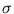and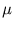,

 p(x) =exp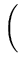-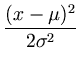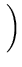. (A.3)

According to the maximum likelihood principle, we will choose the unknown parameters such that the given data are most likely under the obtained distribution. The probability L of the given data set is

 L(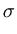,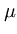) =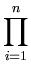p(xi) =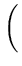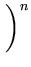exp-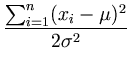. (A.4)

We want to find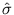and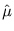that maximize L. Maximizing L is equivalent to maximizing log L, which is also called the log-likelihood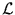,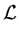(,) = log L(,) = - n log-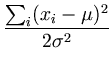+ const . (A.5)

To find the maximum we compute the derivatives of the log-likelihoodand set them to zero: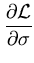= -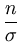+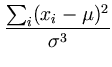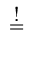0 , (A.6)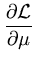=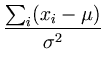0 . (A.7)

Thus, we obtain the values of the parametersand: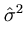=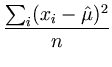, (A.8)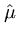=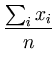. (A.9)

The resulting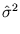is the variance of the distribution andis its center. The extremum ofis indeed a local maximum, as can be seen by computing the Hesse matrix ofand evaluating it at the extreme point (,):

 H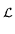=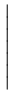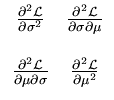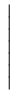, (A.10)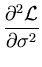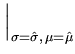=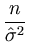-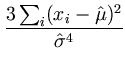=-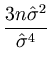= -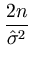, (A.11)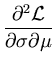=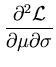= -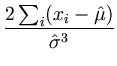= 0 ,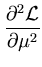= -.

It follows that the Hesse matrix at the extremum is negative definite,

 H|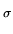=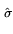,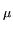=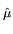=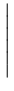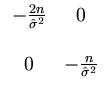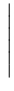. (A.12)

Therefore, the extremum is a local maximum. Moreover, it is also a global maximum. First, for finite parameters, no other extrema exist becauseis a smooth function. Second,is positive for finite parameters, but approaches zero for infinite values. Thus, any maximum must be in the finite range.Next: A.3 Iterative mean Up: A. Statistical tools Previous: A.1 Bayes' theorem
Heiko Hoffmann
2005-03-22